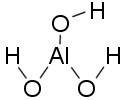## Basic and Amphoteric Hydroxides

Some metal hydroxides are amphoteric, or capable of acting as either an acid or base.

### Learning Objectives

Identify the conditions under which amphoteric hydroxides act as acids.

### Key Takeaways

#### Key Points

• Amphoteric molecules can act as either acids or bases.
• Hydroxides with highly-charged metal cations are often amphoteric.
• Amphoteric hydroxides act as either Bronsted-Lowry bases (accepting protons) or Lewis acids (accepting an electron pair), depending on reaction conditions.

#### Key Terms

• base: a proton acceptor or an electron pair donor
• Lewis acid: an electron pair acceptor

In our discussion of acids and bases so far, hydroxides have always been synonymous with bases. Now we will examine amphoteric hydroxides—that is, hydroxide salts that can act as either an acid or a base, depending on reaction conditions. We will begin with the familiar case of a hydroxide acting as a base.

### Reaction of Amphoteric Hydroxides in Acidic Solution

One of the most common and familiar examples of an amphoteric hydroxide is aluminum hydroxide, Al(OH)3. From our solubility rules, we know that Al(OH)3 is largely insoluble in neutral water; however, in a strongly acidic solution, the situation changes. For example, consider the reaction of Al(OH)3 with HCl:

$\text{HCl}(\text{aq})+\text{Al}(\text{OH})_3(\text{aq})\rightarrow \text{AlCl}_3(\text{aq})+3\text{H}_2\text{O}(\text{l})$

This is a classic acid-base neutralization reaction: the HCl completely protonates all three hydroxides per mole of Al(OH)3, yielding pure water and the salt AlCl3. From what we know about the basic properties of hydroxides so far, this is exactly as we would expect—so how can a hydroxide act as an acid?Aluminum hydroxide: Aluminum hydroxide can act as either a Bronsted-Lowry base, by accepting protons from an acidic solution, or as a Lewis acid, by accepting an electron pair from hydroxide ions in a basic solution.

### Reaction of Amphoteric Hydroxides in Basic Solution

We will now consider aluminum hydroxide’s reaction in a strongly basic solution:

$\text{Al}(\text{OH})_3(\text{aq})+\text{OH}^-(\text{aq})\rightarrow \text{Al}(\text{OH})_4^-(\text{aq})$

Here, aluminum hydroxide picks up an hydroxide ion out of solution, thereby acting as a Lewis acid. How is this possible? Consider the Lewis structure for Al(OH)3.

The central aluminum atom is electron deficient—it forms only three bonds, and the octet rule is not fulfilled; thus, Al3+ is quite happy to accept a pair of electrons and form another bond, given the right conditions. In a basic solution, it forms a bond with an OH ion, pulling it out of solution and lowering the solution’s pH.

### Conclusion

Metal hydroxides with a highly-charged central metal atom can be amphoteric. In addition to aluminum, metals such as zinc, tin, lead, and beryllium can also form amphoteric oxides or hydroxides. Whether such hydroxides behave as acids or bases depends on the surrounding solution’s pH.